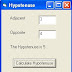# Visual basic Built In Functions with Practice Question

Built In Functions

For the data manipulation, we also have some built-in functions. Some of these are listed below:

Some Numeric Built-in functions:

 Function Comment Sqr (n) Gives the square root of ‘n’ Int (n) Gives the integer part of ‘n’ e.g. Int(2.1) = 2, Int(2.6) = 2. Round (n , x) Rounds a number ‘n’ to ‘x’ decimal places. e.g. Round (6.6666 , 2) = 6.67 Cos (theta) Gives the cosine of theta (theta should be in radians) .We also have other trigonometric functions available. Log (x) Gives the natural log of ‘x’.

Some String Functions:

 Function Comment Left\$ (String , n) Gives the ‘n’ left most characters of the String e.g. Left\$ (“salamander” , 5) = “salam” Right\$ (String , n) Gives the ‘n’ right most characters of the String e.g. Right\$ (“salamander” , 5) = “ander”. Mid\$ (String, n , m) Gives ‘m’ characters starting from the nth character of the string e.g. Mid\$ (“salamander” , 5 , 3) = “man” Len (String) Gives the length of the string e.g. Len (“salam”) = 5 Ucase\$ (String) Outputs the string after converting it into all upper case characters e.g. Ucase\$ (“Salam”) = SALAM Lcase\$ (String) Opposite to the Ucase\$ i.e. converts to all lower case characters.

The aim of this lab exercise is to provide you hands on experience on some of the above mentioned data types and their functions (you can later experiment with others).

Practice Question:

1.      Make a form with 2 labels, 2 text boxes, a picture box and a command button as follows:

2.      This program will be used to calculate the hypotenuse given the two sides of a triangle.

3.      In the click event of the command button write the following code:

Dim opposite As Single
Dim Hypotenuse As Single

Let opposite = Val(Text2.Text)
Let Hypotenuse = Sqr(Adjacent^2 + Opposite^2)
Picture1.Print “The length of the Hypotenuse is”;Hypotenuse

4.      Now run the program and type in different values in the text boxes to test the program.

Practice Question:

1. Make a form as follows:

1. This program will round a positive number to the nearest integer. We are using a built-in function Int(). Int() simply discards the decimal part of positive numbers.

For Example:
Int(2.7) is 2
Int(3) is 3

We will now use this Int() function in ROUNDING NUMBERS and not discarding the decimal part. So if some one enters 2.7 the result should be 3.

1. Write down the following code in the click event of the command button

Rem Declaring two variables n and r
Dim n As Single, r As Single
Let n = Val(Text1.text)
Let r = Int(n+0.5)
Picture1.Print “The rounded value of”; n; “is”; r

1. Now Run the program to test it• Title : Visual basic Built In Functions with Practice Question
• Posted by :
• Date : 11:39
• Labels :